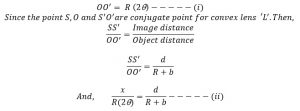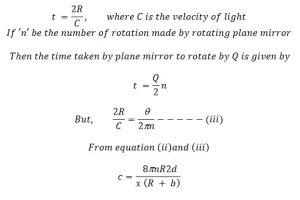# Velocity Of Light By Foucault’s Method

The schematic diagram of Foucault’s method to determine the velocity of light is as shown in figure. It consist of three mirror, such as plane & concave mirror, partially silvered and convex lens as shown in figure. Let us consider a point ‘Q’ on the concave mirror from which light reflected back to rotating mirror. When the plane mirror is in the position m, the rays reflected by it to the lens seems to come on the point ‘o’. Then the image of S is at I such that PO = PQ=R where R is the radius of curvature of concave mirror.

When the plane mirror is rotating at uniform speed is at position of m1 the rays reflected by it to the lens seems to come from O1. If the mirror is rotated by Q the reflected light is rotated by 2Q. from fig,The time taken by light to travel from Q to P and back to Q is,